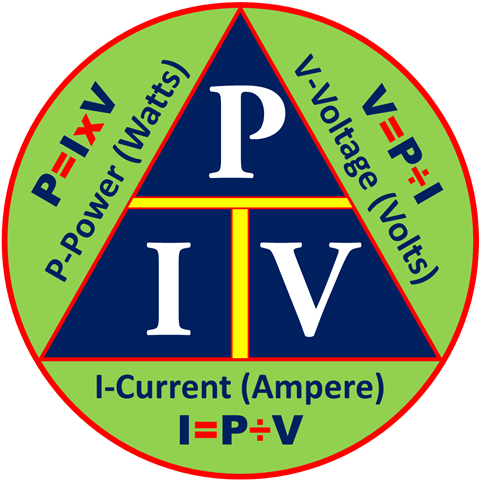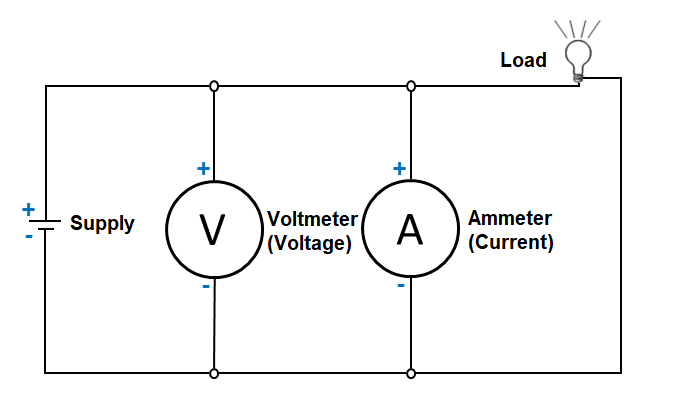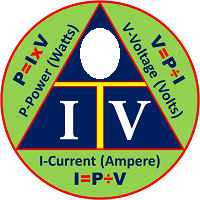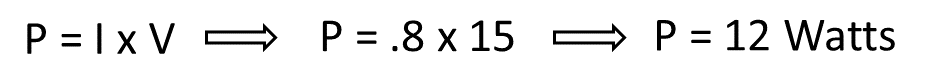## The Power Triangle Formula

The Power, Current, Voltage Formula TriangleHow do we use the formula triangle?

When we use the triangles to solve the unknown values, the given equivalent figure on the apex of the triangle is divided by either of the values at the base of the the triangle, whichever is the given meter reading or electronic value. To find the value of the apex, a given value at either side of the base of the triangle is multiplied by the other base value.

Let’s start now. In this lesson, we will solve for the power consumption with the given values.

The Power Triangle FormulaTo find the power consumption of the load in a circuit, cover the “P” in the triangle and use the resulting equation. Using the circuit above, let us assume that the voltage is 15 volts, and the ammeter reading was 800 milliampere. Calculate the power consumption.Important:
As mentioned earlier, remember to use the correct decimal equivalent when dealing with numbers that are preceded with milli (m), micro (μ) or kilo (k). In this example 800 mA has been used, but was converted to .8 Ampere in the calculation. Otherwise the wrong answer of 12,000 Watts would have come out as a result.

Summary: The electricity formula wheel is a combination or compilation of the different triangle formulas that provides solutions to solving or calculating the resistance, current, voltage and power consumption of an electrical circuit.

When we use the triangles to solve the unknown values, the given equivalent figure on the apex of the triangle is divided by either of the values at the base of the the triangle, whichever is the given meter reading or electronic value. A given value at either side of the base of the triangle is multiplied by the other base value, and vice versa.

Posted in